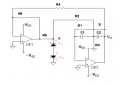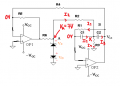# Oscillator circuit

#### xxxyyyba

Joined Aug 7, 2012
289Oscillator circuit is shown.
a) Calculate frequency of oscillations,
b) Calculate minimum value R1 for which circuit still oscillates,
c) Calculate amplitude of voltage VB, for which voltage VA start cuting off

Vz=6.3V, R1=100K, R2=120K, R3=470, R4=2R6=10K, C1=C2=100nF, Vd=0.7V, R4=R5.

I got f_oscillations=232.7Hz and R1_min=250k.
I don't know how to solve task c)
Any idea?

#### Jony130

Joined Feb 17, 2009
5,457
Well, find Vb/Va = gain for Fo and do you know the "cutting off" voltage for this simple diode limiter?
VA start cutting off = "cutting_off voltage" * gain.

#### Jony130

Joined Feb 17, 2009
5,457
Also notice that if R1 is change from 100k to 250k the Fo will also changes.

#### xxxyyyba

Joined Aug 7, 2012
289$$-I_1-I_2+I_3+I_4=0, -\frac{V_B}{R_1}-\frac{V_A-V_X}{R_2}+\frac{V_X-V_B}{\frac{1}{sC_2}}+\frac{V_X}{R_3}=0, \frac{V_B}{R_1}=-\frac{V_X}{\frac{1}{sC_1}}=-sC_1V_X\Rightarrow V_x=-\frac{V_B}{R_1C_1s}, V_X(\frac{1}{R_2}+sC_2+\frac{1}{R_3})-V_B(\frac{1}{R_1}+sC_2)=\frac{V_A}{R_2}, -\frac{V_B}{R_1C_1s}(\frac{1}{R_2}+sC_2+\frac{1}{R_3})-V_B(\frac{1}{R_1}+sC_2)=\frac{V_A}{R_2}, \frac{V_B}{R_1C_1s}(\frac{1}{R_2}+sC_2+\frac{1}{R_3})+V_B(\frac{1}{R_1}+sC_2)=-\frac{V_A}{R_2}$$
And we can find now VB/VA. Is it ok?

Last edited:

#### Jony130

Joined Feb 17, 2009
5,457
Do you manage to find the gain ??
I got this result :

$$\frac{Vb}{Va} = \frac{C1*R1}{(C1+C2)*R2)} = \frac{25}{24} = 1.041\frac{V}{V}$$

And Fo is around 147Hz

Also as a side note I immediately recognized this circuit with OP2 alone, as is used in guitar effects
http://www.muzique.com/news/wahantiwah-mod/

#### xxxyyyba

Joined Aug 7, 2012
289
I definitely made mistake somewhere. Your gain Vb/Va doesn't depend on s...

#### xxxyyyba

Joined Aug 7, 2012
289
Jony30,
I got same result as you in post #5 using analysis in post #4.

#### Jony130

Joined Feb 17, 2009
5,457
Good for you.
And the transfer function (if i do not make any error) in standard form is :
$$\frac{V_b}{V_a} =-\frac{ \frac{1}{C2 R2}s } { s^2+\frac{(C1+C2)}{C1C2R1}s\frac{R2+R3}{C1C2R1R2R3}[\tex]$$

T(s) = (a1*s)/(s^2 + ωo/Q + ωo^2)

And the gain at Fo is

Av =(a1*Q)/ωo = (C1 R1)/((C1 + C2) R2)